# CNIT 141: 9. Elliptic Curve Cryptosystems

11. Nov 2017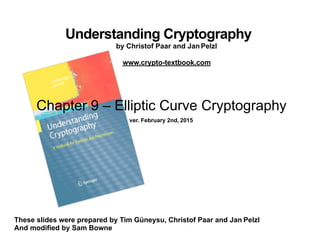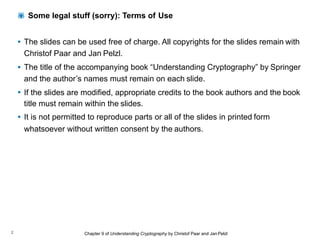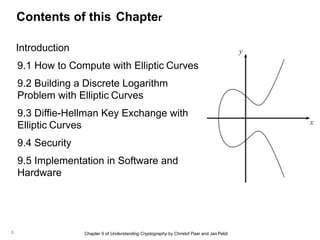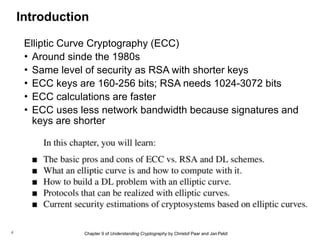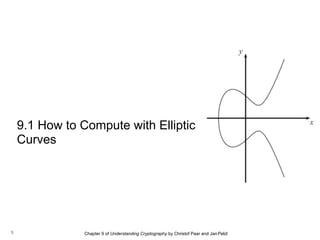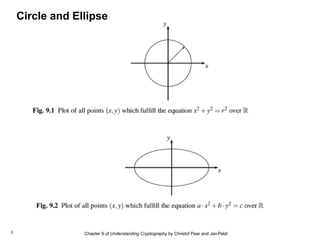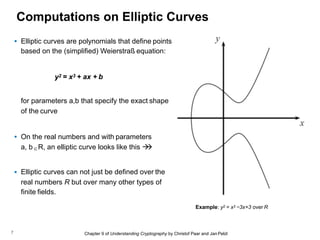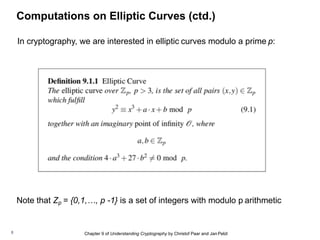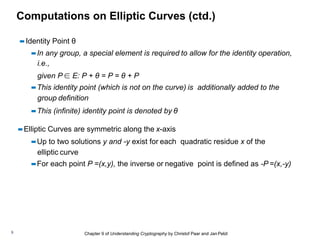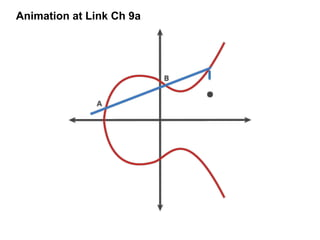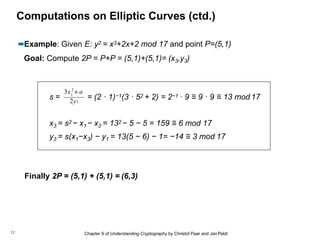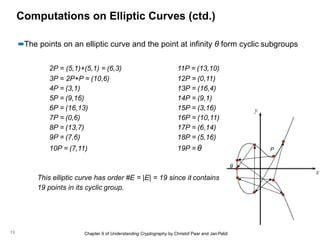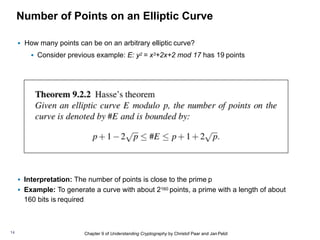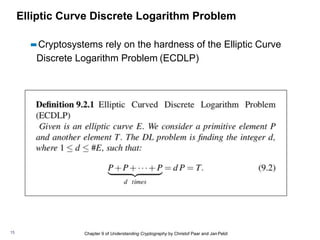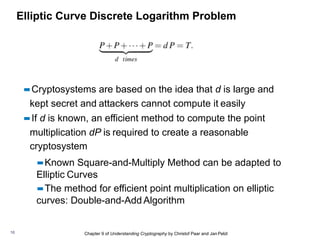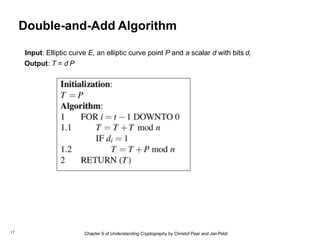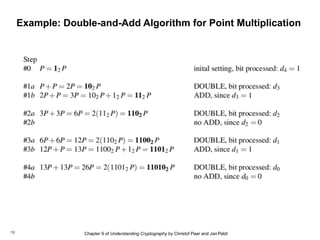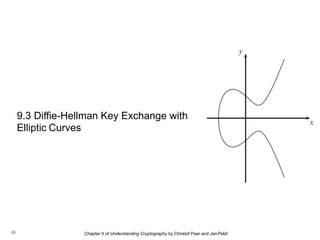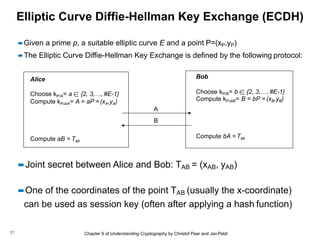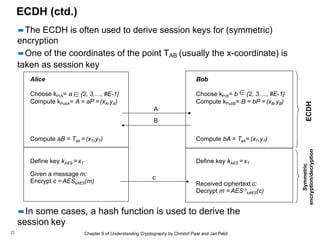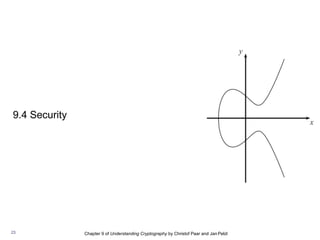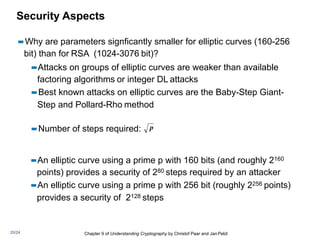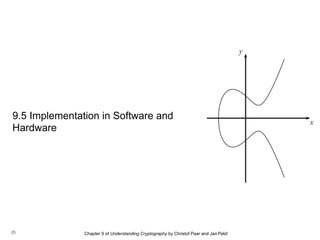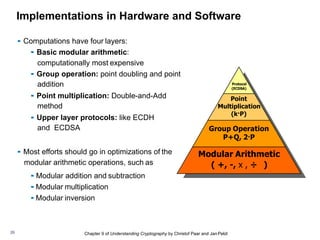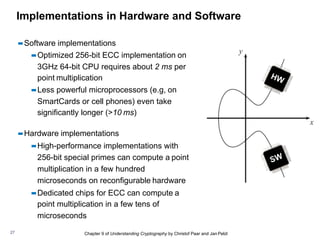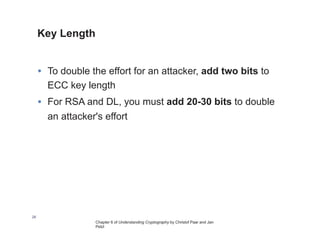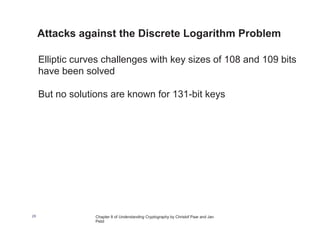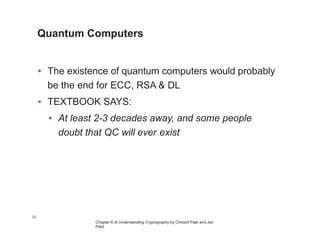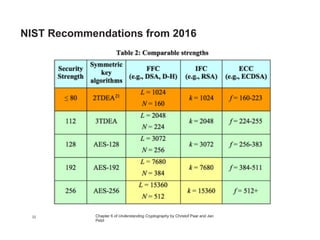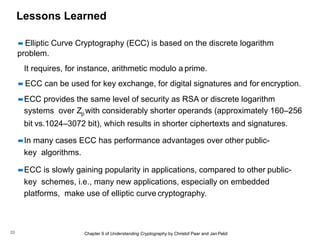1 von 34

### CNIT 141: 9. Elliptic Curve Cryptosystems

• 1. Understanding Cryptography by Christof Paar and Jan Pelzl www.crypto-textbook.com These slides were prepared by Tim Güneysu, Christof Paar and Jan Pelzl And modified by Sam Bowne Chapter 9 – Elliptic Curve Cryptography ver. February 2nd, 2015
• 2. Chapter 9 of Understanding Cryptography by Christof Paar and Jan Pelzl2 • The slides can be used free of charge. All copyrights for the slides remain with Christof Paar and Jan Pelzl. • The title of the accompanying book “Understanding Cryptography” by Springer and the author’s names must remain on each slide. • If the slides are modified, appropriate credits to the book authors and the book title must remain within the slides. • It is not permitted to reproduce parts or all of the slides in printed form whatsoever without written consent by the authors. ! Some legal stuff (sorry): Terms of Use
• 3. Chapter 9 of Understanding Cryptography by Christof Paar and Jan Pelzl Contents of this Chapter Introduction 9.1 How to Compute with Elliptic Curves 9.2 Building a Discrete Logarithm Problem with Elliptic Curves 9.3 Diffie-Hellman Key Exchange with Elliptic Curves 9.4 Security 9.5 Implementation in Software and Hardware 3
• 4. Chapter 9 of Understanding Cryptography by Christof Paar and Jan Pelzl Elliptic Curve Cryptography (ECC) • Around sinde the 1980s • Same level of security as RSA with shorter keys • ECC keys are 160-256 bits; RSA needs 1024-3072 bits • ECC calculations are faster • ECC uses less network bandwidth because signatures and keys are shorter 4 Introduction
• 5. Chapter 9 of Understanding Cryptography by Christof Paar and Jan Pelzl 9.1 How to Compute with Elliptic Curves 5
• 6. Chapter 9 of Understanding Cryptography by Christof Paar and Jan Pelzl6 Circle and Ellipse
• 7. Chapter 9 of Understanding Cryptography by Christof Paar and Jan Pelzl Computations on Elliptic Curves Example: y2 = x3 −3x+3 over R 7 • Elliptic curves are polynomials that define points based on the (simplified) Weierstraß equation: y2 = x3 + ax + b for parameters a,b that specify the exact shape of the curve • On the real numbers and with parameters a, b ∈R, an elliptic curve looks like this !! • Elliptic curves can not just be defined over the real numbers R but over many other types of finite fields.
• 8. Chapter 9 of Understanding Cryptography by Christof Paar and Jan Pelzl Computations on Elliptic Curves (ctd.) 8 In cryptography, we are interested in elliptic curves modulo a prime p: Note that Zp = {0,1,…, p -1} is a set of integers with modulo p arithmetic
• 9. Chapter 9 of Understanding Cryptography by Christof Paar and Jan Pelzl Computations on Elliptic Curves (ctd.) ▪▪Identity Point θ ▪▪In any group, a special element is required to allow for the identity operation, i.e., given P ∈ E: P + θ = P = θ + P ▪▪This identity point (which is not on the curve) is additionally added to the group definition ▪▪This (infinite) identity point is denoted by θ ▪▪Elliptic Curves are symmetric along the x-axis ▪▪Up to two solutions y and -y exist for each quadratic residue x of the elliptic curve ▪▪For each point P =(x,y), the inverse or negative point is defined as -P =(x,-y) 9
• 10. Computations on Elliptic Curves (ctd.) ▪▪Generating a group of points on elliptic curves based on point addition operation P+Q = R, i.e., (xP,yP) + (xQ,yQ) = (xR,yR) ▪▪Geometric Interpretation of point addition operation ▪▪Draw straight line through P and Q; if P=Q use tangent line instead ▪▪Mirror third intersection point of drawn line with the elliptic curve along the x-axis PointAddition Point Doubling Chapter 9 of Understanding Cryptography by Christof Paar and Jan Pelzl10/24 =P+P
• 11. Animation at Link Ch 9a
• 12. Chapter 9 of Understanding Cryptography by Christof Paar and Jan Pelzl Computations on Elliptic Curves (ctd.) ▪▪Example: Given E: y2 = x3+2x+2 mod 17 and point P=(5,1) Goal: Compute 2P = P+P = (5,1)+(5,1)= (x3,y3) s = = (2 · 1)−1(3 · 52 + 2) = 2−1 · 9 ≡ 9 · 9 ≡ 13 mod 17 x3 = s2 − x1 − x2 = 132 − 5 − 5 = 159 ≡ 6 mod 17 y3 = s(x1−x3) − y1 = 13(5 − 6) − 1= −14 ≡ 3 mod 17 Finally 2P = (5,1) + (5,1) = (6,3) 2 1 2y1 3x + a 12
• 13. Chapter 9 of Understanding Cryptography by Christof Paar and Jan Pelzl Computations on Elliptic Curves (ctd.) ▪▪The points on an elliptic curve and the point at infinity θ form cyclic subgroups 2P = (5,1)+(5,1) = (6,3) 3P = 2P+P = (10,6) 4P = (3,1) 5P = (9,16) 6P = (16,13) 7P = (0,6) 8P = (13,7) 9P = (7,6) 10P = (7,11) 11P = (13,10) 12P = (0,11) 13P = (16,4) 14P = (9,1) 15P = (3,16) 16P = (10,11) 17P = (6,14) 18P = (5,16) 19P = θ P θ This elliptic curve has order #E = |E| = 19 since it contains 19 points in its cyclic group. 13
• 14. Chapter 9 of Understanding Cryptography by Christof Paar and Jan Pelzl Number of Points on an Elliptic Curve • How many points can be on an arbitrary elliptic curve? • Consider previous example: E: y2 = x3+2x+2 mod 17 has 19 points • Interpretation: The number of points is close to the prime p • Example: To generate a curve with about 2160 points, a prime with a length of about 160 bits is required 14
• 15. Chapter 9 of Understanding Cryptography by Christof Paar and Jan Pelzl Elliptic Curve Discrete Logarithm Problem ▪▪Cryptosystems rely on the hardness of the Elliptic Curve Discrete Logarithm Problem (ECDLP) 15
• 16. Chapter 9 of Understanding Cryptography by Christof Paar and Jan Pelzl Elliptic Curve Discrete Logarithm Problem ▪▪Cryptosystems are based on the idea that d is large and kept secret and attackers cannot compute it easily ▪▪If d is known, an efficient method to compute the point multiplication dP is required to create a reasonable cryptosystem ▪▪Known Square-and-Multiply Method can be adapted to Elliptic Curves ▪▪The method for efficient point multiplication on elliptic curves: Double-and-Add Algorithm 16
• 17. Chapter 9 of Understanding Cryptography by Christof Paar and Jan Pelzl Double-and-Add Algorithm Input: Elliptic curve E, an elliptic curve point P and a scalar d with bits di Output: T = d P 17
• 18. Chapter 9 of Understanding Cryptography by Christof Paar and Jan Pelzl Example: Double-and-Add Algorithm for Point Multiplication 18
• 20. Chapter 9 of Understanding Cryptography by Christof Paar and Jan Pelzl 9.3 Diffie-Hellman Key Exchange with Elliptic Curves 20
• 21. Chapter 9 of Understanding Cryptography by Christof Paar and Jan Pelzl Elliptic Curve Diffie-Hellman Key Exchange (ECDH) ▪▪Given a prime p, a suitable elliptic curve E and a point P=(xP,yP) ▪▪The Elliptic Curve Diffie-Hellman Key Exchange is defined by the following protocol: ▪▪Joint secret between Alice and Bob: TAB = (xAB, yAB) ▪▪One of the coordinates of the point TAB (usually the x-coordinate) can be used as session key (often after applying a hash function) Alice Compute aB = Tab Choose kPrA= a ∈ {2, 3,…, #E-1} Compute kPubA= A = aP =(xA,yA) Bob Compute bA = Tab A B 21 Choose kPrB= b ∈ {2, 3,…, #E-1} Compute kPubB= B = bP = (xB,yB)
• 22. Chapter 9 of Understanding Cryptography by Christof Paar and Jan Pelzl ECDH (ctd.) ▪▪The ECDH is often used to derive session keys for (symmetric) encryption ▪▪One of the coordinates of the point TAB (usually the x-coordinate) is taken as session key ▪▪In some cases, a hash function is used to derive the session key Alice Compute aB = Tab =(xT,yT) Define key kAES =xT Given a message m: Encrypt c =AESkAES(m) Choose kPrA= a ∈ {2, 3,…, #E-1} Compute kPubA= A = aP =(xA,yA) Compute bA = Tab= (xT,yT) Define key kAES =xT Received ciphertext c: Decrypt m = AES-1 kAES(c) A B Bob Choose kPrB= b ∈ {2, 3,…, #E-1} Compute kPubB= B = bP = (xB,yB) c ECDH 22 Symmetric encryption/decryption
• 23. Chapter 9 of Understanding Cryptography by Christof Paar and Jan Pelzl 9.4 Security 23
• 24. Chapter 9 of Understanding Cryptography by Christof Paar and Jan Pelzl Security Aspects ▪▪Why are parameters signficantly smaller for elliptic curves (160-256 bit) than for RSA (1024-3076 bit)? ▪▪Attacks on groups of elliptic curves are weaker than available factoring algorithms or integer DL attacks ▪▪Best known attacks on elliptic curves are the Baby-Step Giant- Step and Pollard-Rho method ▪▪Number of steps required: ▪▪An elliptic curve using a prime p with 160 bits (and roughly 2160 points) provides a security of 280 steps required by an attacker ▪▪An elliptic curve using a prime p with 256 bit (roughly 2256 points) provides a security of 2128 steps p 20/24
• 25. Chapter 9 of Understanding Cryptography by Christof Paar and Jan Pelzl 9.5 Implementation in Software and Hardware 25
• 26. Chapter 9 of Understanding Cryptography by Christof Paar and Jan Pelzl Implementations in Hardware and Software ▪▪ Computations have four layers: ▪▪ Basic modular arithmetic: computationally most expensive ▪▪ Group operation: point doubling and point addition ▪▪ Point multiplication: Double-and-Add method ▪▪ Upper layer protocols: like ECDH and ECDSA ▪▪ Most efforts should go in optimizations of the modular arithmetic operations, such as ▪▪ Modular addition and subtraction ▪▪ Modular multiplication ▪▪ Modular inversion Protocol (ECDSA) Point Multiplication (k·P) Group Operation P+Q, 2·P Modular Arithmetic ( +, -, x , ÷ ) 26
• 27. Chapter 9 of Understanding Cryptography by Christof Paar and Jan Pelzl Implementations in Hardware and Software ▪▪Software implementations ▪▪Optimized 256-bit ECC implementation on 3GHz 64-bit CPU requires about 2 ms per point multiplication ▪▪Less powerful microprocessors (e.g, on SmartCards or cell phones) even take significantly longer (>10 ms) ▪▪Hardware implementations ▪▪High-performance implementations with 256-bit special primes can compute a point multiplication in a few hundred microseconds on reconfigurable hardware ▪▪Dedicated chips for ECC can compute a point multiplication in a few tens of microseconds 27
• 28. Chapter 6 of Understanding Cryptography by Christof Paar and Jan Pelzl Key Length 28 • To double the effort for an attacker, add two bits to ECC key length • For RSA and DL, you must add 20-30 bits to double an attacker's effort
• 29. Chapter 8 of Understanding Cryptography by Christof Paar and Jan Pelzl 29 Attacks against the Discrete Logarithm Problem Elliptic curves challenges with key sizes of 108 and 109 bits have been solved But no solutions are known for 131-bit keys
• 30. Chapter 6 of Understanding Cryptography by Christof Paar and Jan Pelzl Quantum Computers 30 • The existence of quantum computers would probably be the end for ECC, RSA & DL • TEXTBOOK SAYS: • At least 2-3 decades away, and some people doubt that QC will ever exist
• 31. Chapter 6 of Understanding Cryptography by Christof Paar and Jan Pelzl 31 NIST Recommendations from 2016
• 32. Chapter 6 of Understanding Cryptography by Christof Paar and Jan Pelzl 32 NIST Recommendations from 2016
• 33. Chapter 9 of Understanding Cryptography by Christof Paar and Jan Pelzl33 ▪▪ Elliptic Curve Cryptography (ECC) is based on the discrete logarithm problem. It requires, for instance, arithmetic modulo a prime. ▪▪ ECC can be used for key exchange, for digital signatures and for encryption. ▪▪ECC provides the same level of security as RSA or discrete logarithm systems over Zp with considerably shorter operands (approximately 160–256 bit vs.1024–3072 bit), which results in shorter ciphertexts and signatures. ▪▪In many cases ECC has performance advantages over other public- key algorithms. ▪▪ECC is slowly gaining popularity in applications, compared to other public- key schemes, i.e., many new applications, especially on embedded platforms, make use of elliptic curve cryptography. Lessons Learned